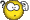# Two's Complement MisunderstandingClassicListThreaded4 messagesOpen this post in threaded view
|

## Two's Complement Misunderstanding

 Hi, In the book (page 31) two's complement of x is said to be calculated by (2^n)-x. It then goes on to say that to calculate -2 you perform the following operation: (2^4)-2. However, why if x=-2 in this case is it (2^4)-2 and not (2^4)-(-2)? Also, to calculate two's complement where x=2, one would perform the operation: (2^4)-(-2) in order to yield the result 0010. I am very confused...Can anyone explain? Thanks.
Open this post in threaded view
|

## Re: Two's Complement Misunderstanding

 Administrator It's those pesky minus signs, curse of Algebra students worldwide...  Also, there are too many twos to keep straight; I'm going to use 3. The binary representation of -3 is the two's complement of +3. The 4-bit two's complement of +3 is 2^4 - (+3) which is 13, so -3 is 1101 in 4-bit binary. Overly picky mathematicians will tell us that two's complements only exist for positive numbers, but we're programmers and engineers so we don't listen to them. The 4-bit two's complement of -3 is 2^4 - (-3) = 19 = 10011 and we throw away the overflow and get 0011, the 4-bit binary representation of +3. --Mark